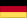Findings
of the static analysis of the arch dam Karakaya in Turkey
by Dr. sc. techn. ETH Rudolf Trinkner

# Method of Calculation and Theory

The calculations were made by a computer program based on FLASH2 and its continued de-velopment. The program was used for recalculations of various existing very large arch dams. The results of the recalculations and studies of these structures were highly concordant. The finite element method was used for the static evidence.

The finite element method can be defined as follows:

• The continuum is divided into a finite number of elements whose behaviour is described by a certain number of parameters, and
• the solution of the overall system, as an accumulation of elements, strictly follows the rules applied to other standard discretisation problems.

The finite element method assumes that the mechanical behaviour of a continuously curved shell can be adequately emulated using a surface section of a polyhedron comprised of small triangles. Intuitively, it would seem that continuous refinement of the subdivision must lead to convergence. Indeed, experiments have confirmed this. This convergence has also been mathematically proven ("Arch Dams analysed by a linear finite element shell solution pro-gram"; O. C. Zienkiewicz and others).

Contrary to international concrete dam construction conventions this program uses negative signs to signify compressive stress and positive signs to signify tensile stress.